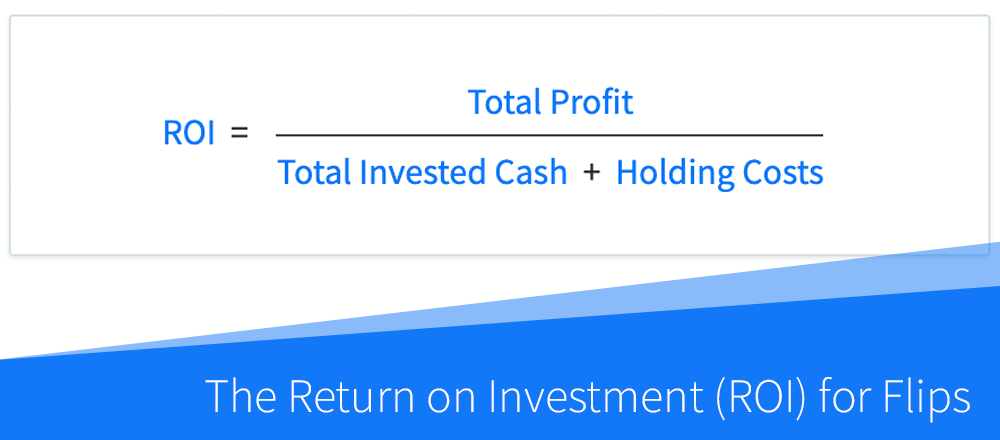# DealCheck Blog - Real Estate Analysis

Learn how to calculate and use the most important property analysis metrics.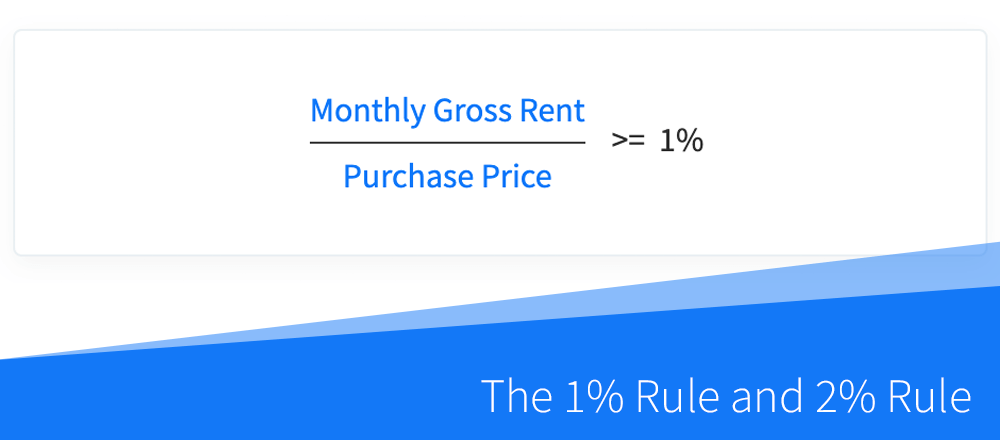## How to Use the 1% Rule and 2% Rule in Real Estate Investing

The 1% Rule and 2% Rule are often used by real estate investors as purchase and investment criteria when evaluating rental properties and BRRRR's prior to their purchase. They can also be used as general valuation criteria when comparing the affordability of...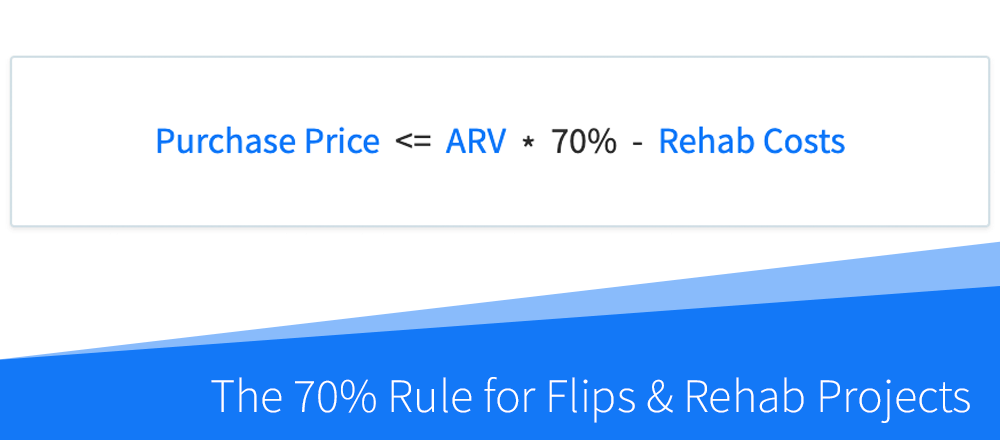## What Is the 70% Rule in Real Estate and How Is It Useful When Making Offers?

The so-called 70% Rule is commonly used by real estate investors, house flippers and wholesalers as a quick rule of thumb to calculate offer prices on flips, BRRRR's, wholesale deals, and other projects that require rehab work. Although the rationale behind this rule...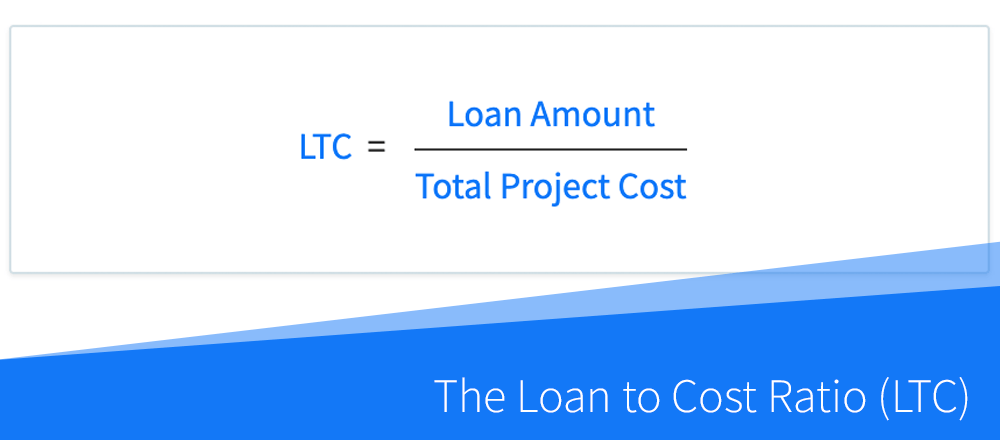## What Is the Loan to Cost Ratio (LTC) And How Is It Used by Lenders?

The loan to cost ratio (LTC) is often used by hard money and commercial real estate lenders when underwriting loans for flips, BRRRR's and new construction projects. Simply put, it shows the percentage of a project's total cost that is financed by the lender. For...## Understanding the Capitalization Rate (Cap Rate) for Rental Properties

The capitalization rate, commonly referred to simply as the cap rate, is a popular rate of return that is used when evaluating and comparing rental properties, especially when working with commercial real estate. Calculated by dividing a property's net operating...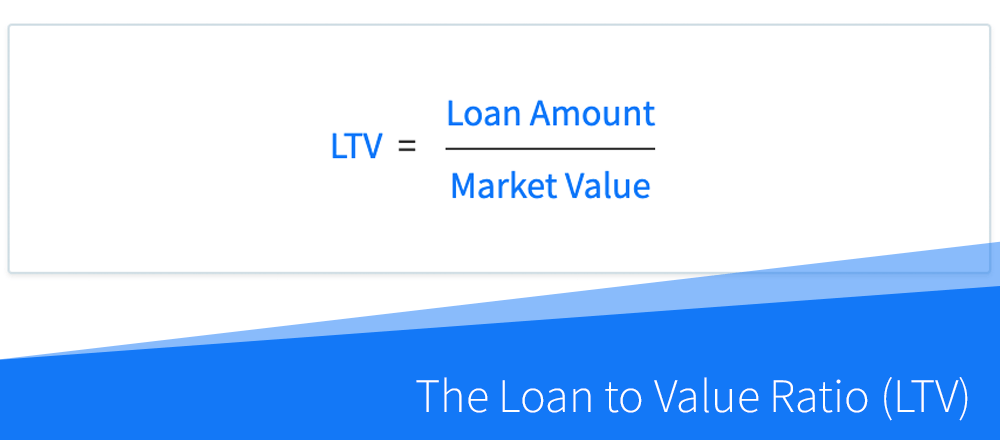## What Is the Loan to Value Ratio (LTV) And How Is It Used by Lenders?

The loan to value ratio (LTV) is one of the primary metrics used by real estate lenders when underwriting loans for rental properties, BRRRR's and flips. Simply put, it shows the percentage of a property's value that is taken up by the loan amount. For lenders, it is...## How to Calculate the Internal Rate of Return (IRR) for Rental Properties

Note: This article describes the IRR calculation for rental properties. To learn about the annualized ROI for flips and rehab projects, read this article instead. The internal rate of return, abbreviated as IRR, is a widely used metric of profitability and a measure...## What Is the Equity Multiple in Real Estate and How Is It Calculated?

The equity multiple is a measure of profitability and investment return commonly used by commercial real estate investors, although it can be used as a performance metric when analyzing and comparing any rental property or BRRRR. Equity multiple takes into account the...## How to Calculate the Return on Investment (ROI) for Rental Properties

Note: This article describes the ROI calculation specifically for rental properties, and not flips and rehab projects, which is calculated differently. The return on investment, abbreviated as ROI, is one of the primary metrics of profitability and a measure of the...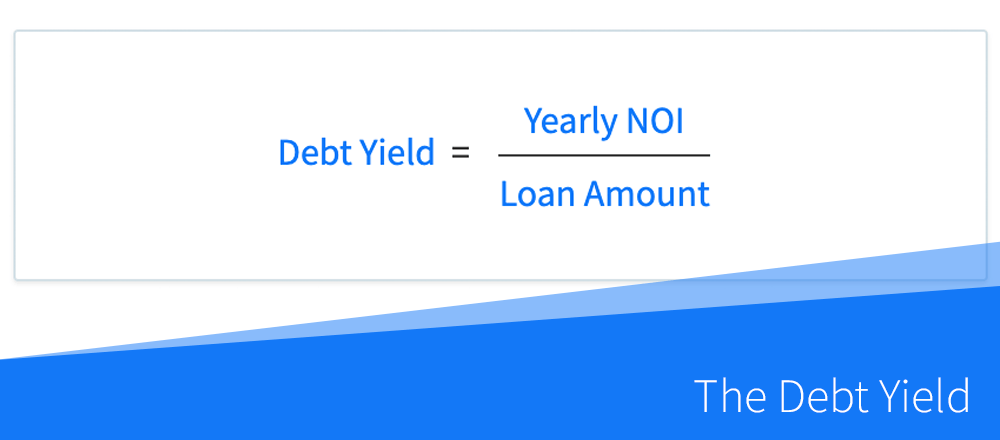## How to Calculate the Debt Yield in Commercial Real Estate

The debt yield is an indicator of leverage and loan risk that is often used by real estate lenders when underwriting loans for rental properties, especially when working with commercial real estate. In simple terms, debt yield can be used to estimate how long it will...